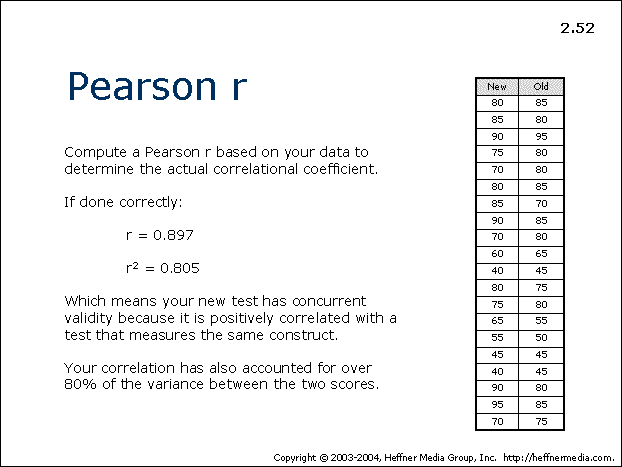# 52: Pearson r: Actual Correlational Coefficient## Pearson r

• Compute a Pearson r based on your data to determine the actual correlational coefficient.
• If done correctly:
• r = 0.897
• r² = 0.805
• Which means your new test has concurrent validity because it is positively correlated with a test that measures the same construct.
• Your correlation has also accounted for over 80% of the variance between the two scores.